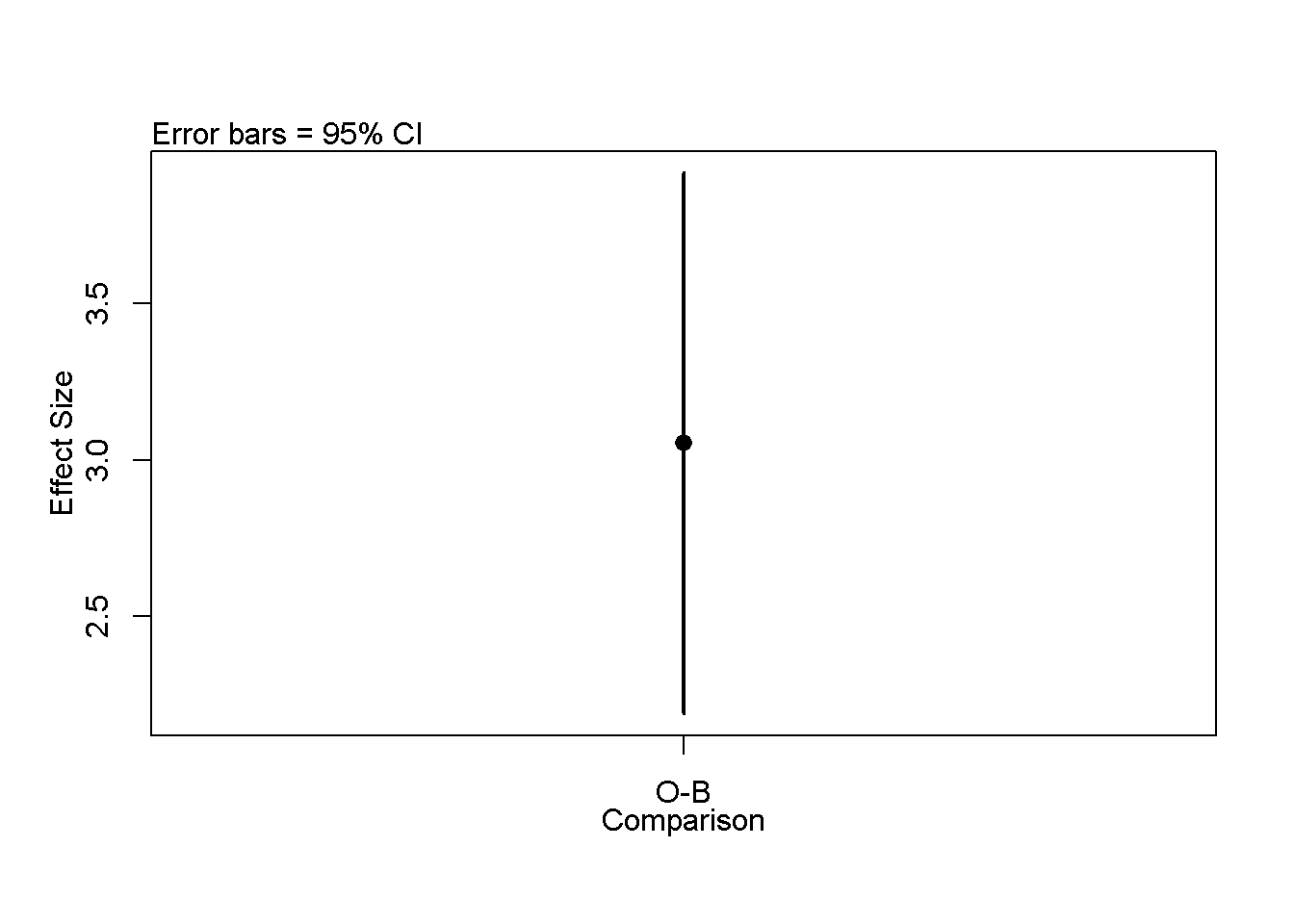Changes to this code will be announced via email and the course FaceBook page CalU EcoStats.

For more labs and tutorials see my WordPress Site lo.brow.R

# 1 Function plotTukeysHSD()

## 1.1 Purpose

This function takes the output of the TukeyHSD() function and plots the estimated differences and confidence intervals. It is an alternative to the output of the plot() function when called on an object produced by the TukeyHSD(). In particular it

• plots different comparisons along the x-axis
• automatically add a label that the error bars are 95%CIs

## 1.2 On Tukey’s HSD and multiple comparisons

Please note that there is considerable discussion about the merits of different approaches to multiple comparisons. Many researchers currently advocate controling the False Discovery Rate rather than the family-wise error rate as is done with Tukey’s HSD. Moreover, there are many reasons to question the use of p-values, significance thresholds, and null hypothesis statistical testing (NHST) in the first place.

For a thoughtful perspective on the use of multiple comparisons procedures in ecology, see Ruxton and Beauchamp 2008. Time for some a priori thinking about post hoc tesing. For an introduction to False Discovery Rate control for ecologists, see Verhoeven et al. 2005. Implementing false discovery rate control: increasing your power

# 2 plotTukeysHSD() Code

# means = 3 mean values calcualted from raw data
# se = 3 standard errors
# categories = 3 categorical / factors that group the data
# x.axis.label = what should be plotted on the x axis
# y.axis.label = what should be plotted on the y axis

#### The function STARTS here ####
plotTukeyHSD <- plotTukeysHSD <- function(tukey.out,
x.axis.label = "Comparison",
y.axis.label = "Effect Size",

tukey.out <- as.data.frame(tukey.out[])
means <- tukey.out$diff categories <- row.names(tukey.out) groups <- length(categories) ci.low <- tukey.out$lwr
ci.up  <- tukey.out$upr n.means <- length(means) #determine where to plot points along x-axis x.values <- 1:n.means x.values <- x.values/adjust.x.spacing # calculate values for plotting limits y.max <- max(ci.up) + max(ci.up)*axis.adjust y.min <- min(ci.low) - max(ci.low)*axis.adjust if(groups == 2){ x.values <- c(0.25, 0.5)} if(groups == 3){ x.values <- c(0.25, 0.5,0.75)} x.axis.min <- min(x.values)-0.05 x.axis.max <- max(x.values)+0.05 x.limits <- c(x.axis.min,x.axis.max) #Plot means plot(means ~ x.values, xlim = x.limits, ylim = c(y.min,y.max), xaxt = "n", xlab = "", ylab = "", cex = 1.25, pch = 16) axis(side = 1, at = x.values, labels = categories, ) #Plot upper error bar lwd. <- 2 arrows(y0 = means, x0 = x.values, y1 = ci.up, x1 = x.values, length = 0, lwd = lwd.) #Plot lower error bar arrows(y0 = means, x0 = x.values, y1 = ci.low, x1 = x.values, length = 0, lwd = lwd.) #add reference line at 0 abline(h = 0, col = 2, lwd = 2, lty =2) mtext(text = x.axis.label,side = 1,line = 1.75) mtext(text = y.axis.label,side = 2,line = 1.95) mtext(text = "Error bars = 95% CI",side = 3,line = 0,adj = 0) } #### The function ENDS here #### #### The function ENDS here #### #### The function ENDS here #### #### The function ENDS here #### #### The function ENDS here #### # 3 Test the function THIS CODE IS NOT PART OF THE FUNCTION ## 3.1 Test the function on data w/3 groups Calcualte mean and SE from Fisher’s iris data. (This is code to test the function and not part of the function) ### 3.1.1 Fit ANOVA model w/ aov() #(This is code to test the function and not part of the function) data(iris) iris.aov <- aov(Sepal.Length ~ Species, data = iris) ### 3.1.2 Calcualte p-values etc w/ TukeyHSD() iris.Tukey <- TukeyHSD(iris.aov) ### 3.1.3 Compare default plot with plotTukeysHSD() #(This is code to test the function and not part of the function) par(mfrow = c(1,2)) plot(iris.Tukey) plotTukeysHSD(iris.Tukey)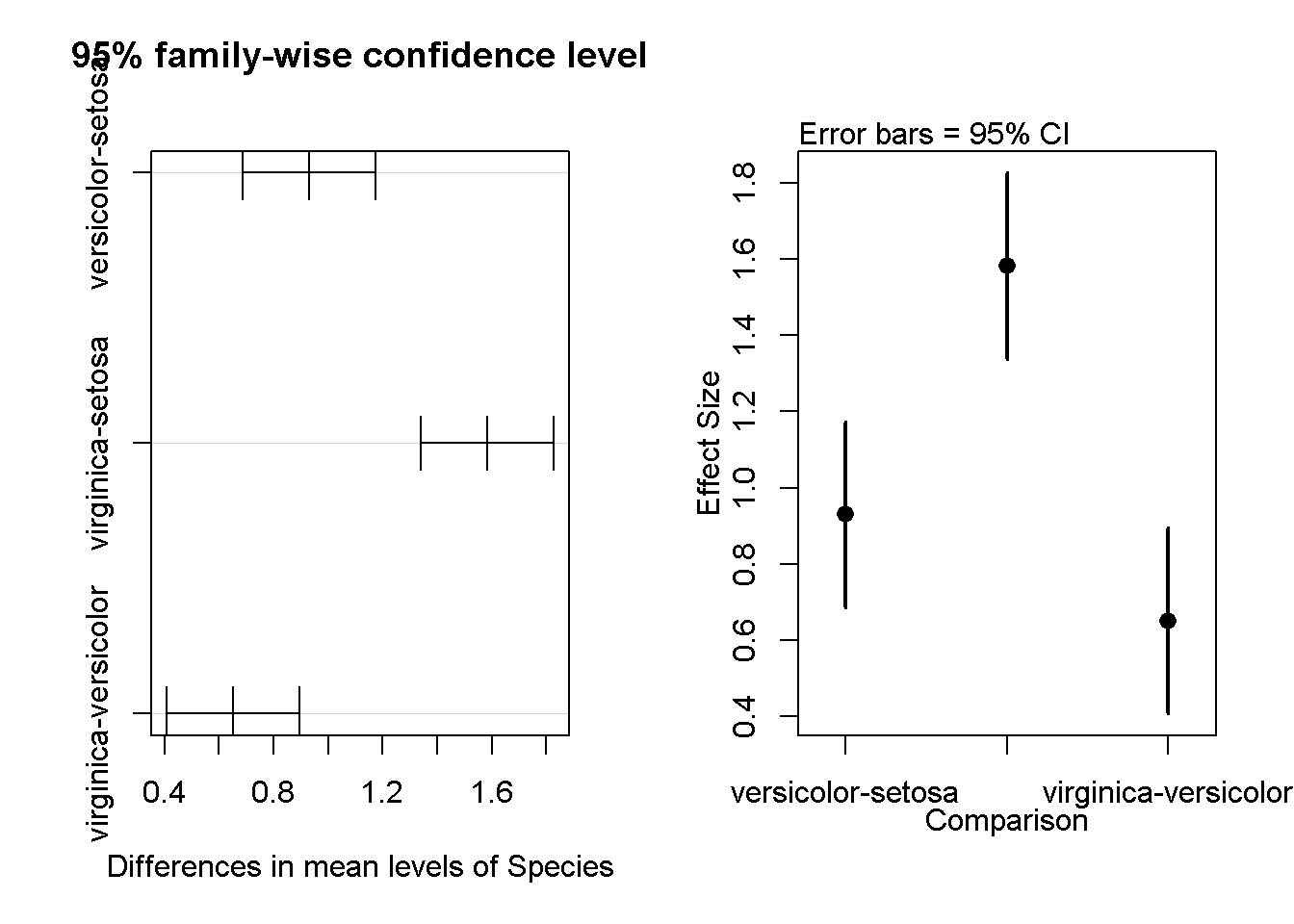## Test the function on data w/4 groups #(This is code to test the function and not part of the function) library(MASS) data("crabs") #make 4 sep groups crabs$groups.4 <- with(crabs, paste(sp,sex,sep = "."))

crab.aov <- aov(FL ~ groups.4, data = crabs)

crab.Tukey <- TukeyHSD(crab.aov)

plot(crab.Tukey)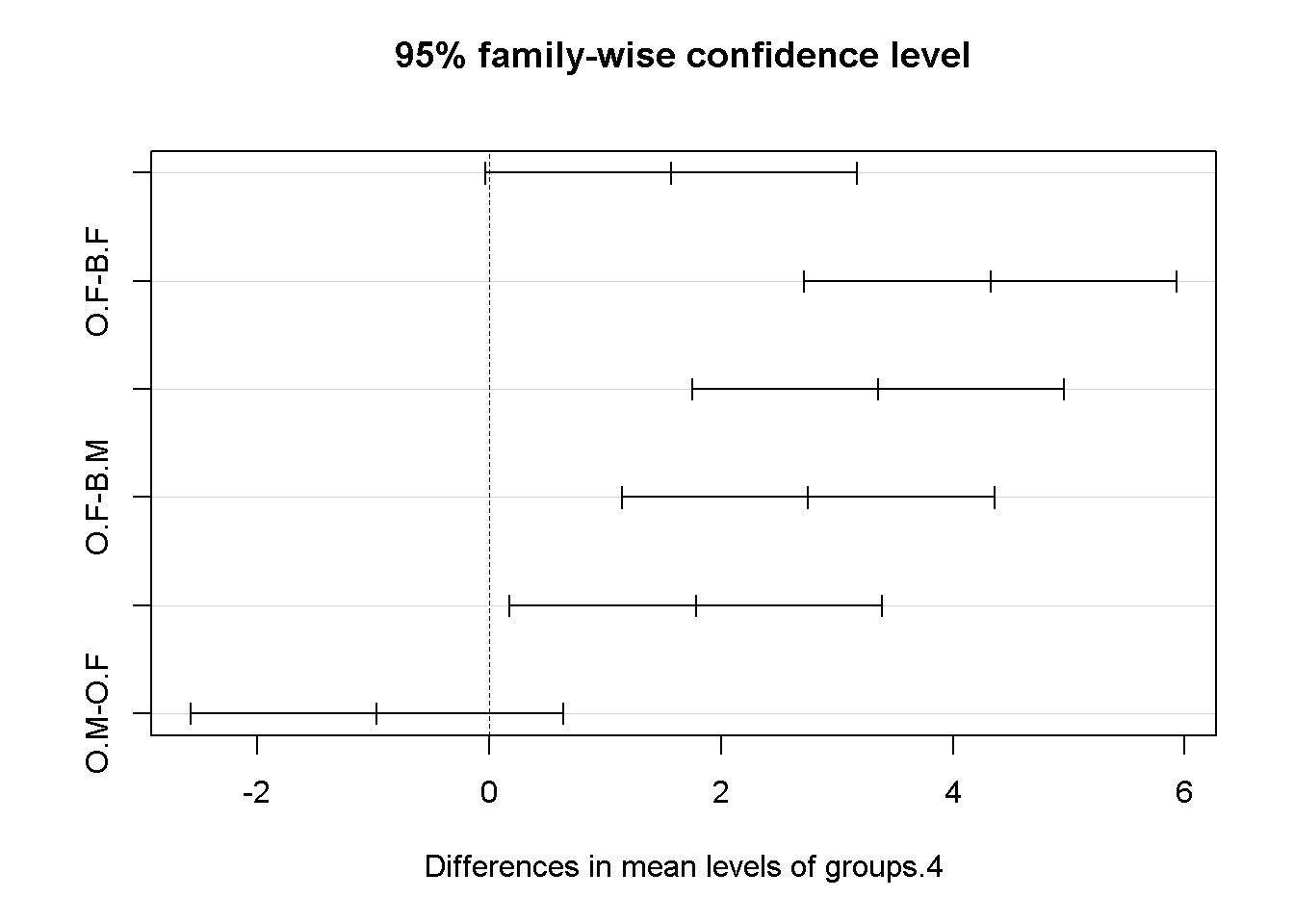plotTukeysHSD(crab.Tukey)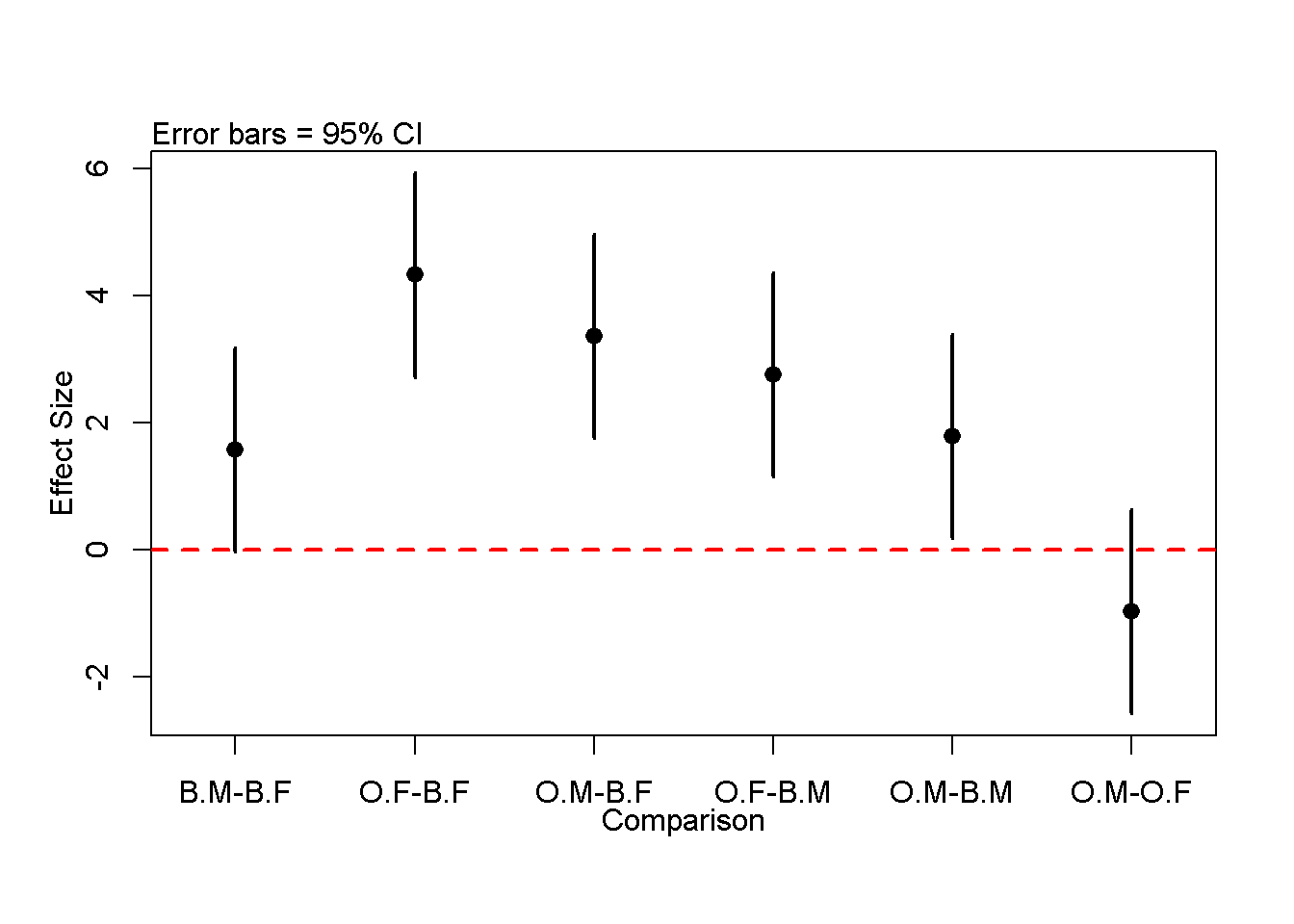# 4 To Do

• Make it work with multi-way anova objects (see problem belw)
• Prevent comparisons labels from over running

## 4.1 Problem to fix

When a multi-way ANOVA is runk the TukeyHSD() output is a list. The function needs to know to go to the correct element of the list.

crab.aov <- aov(FL ~ sp*sex, data = crabs)

crab.Tukey <- TukeyHSD(crab.aov)

plot(crab.Tukey)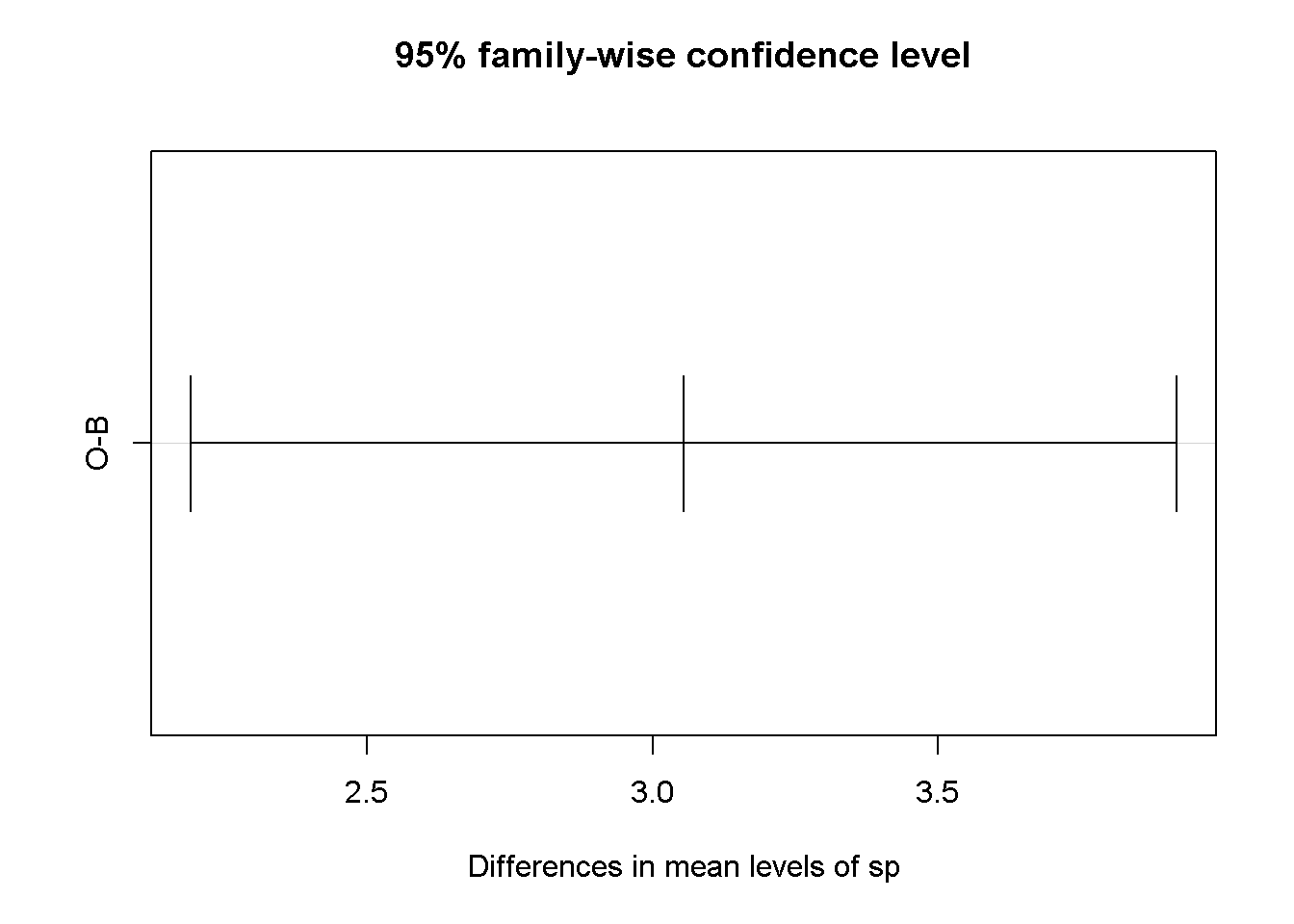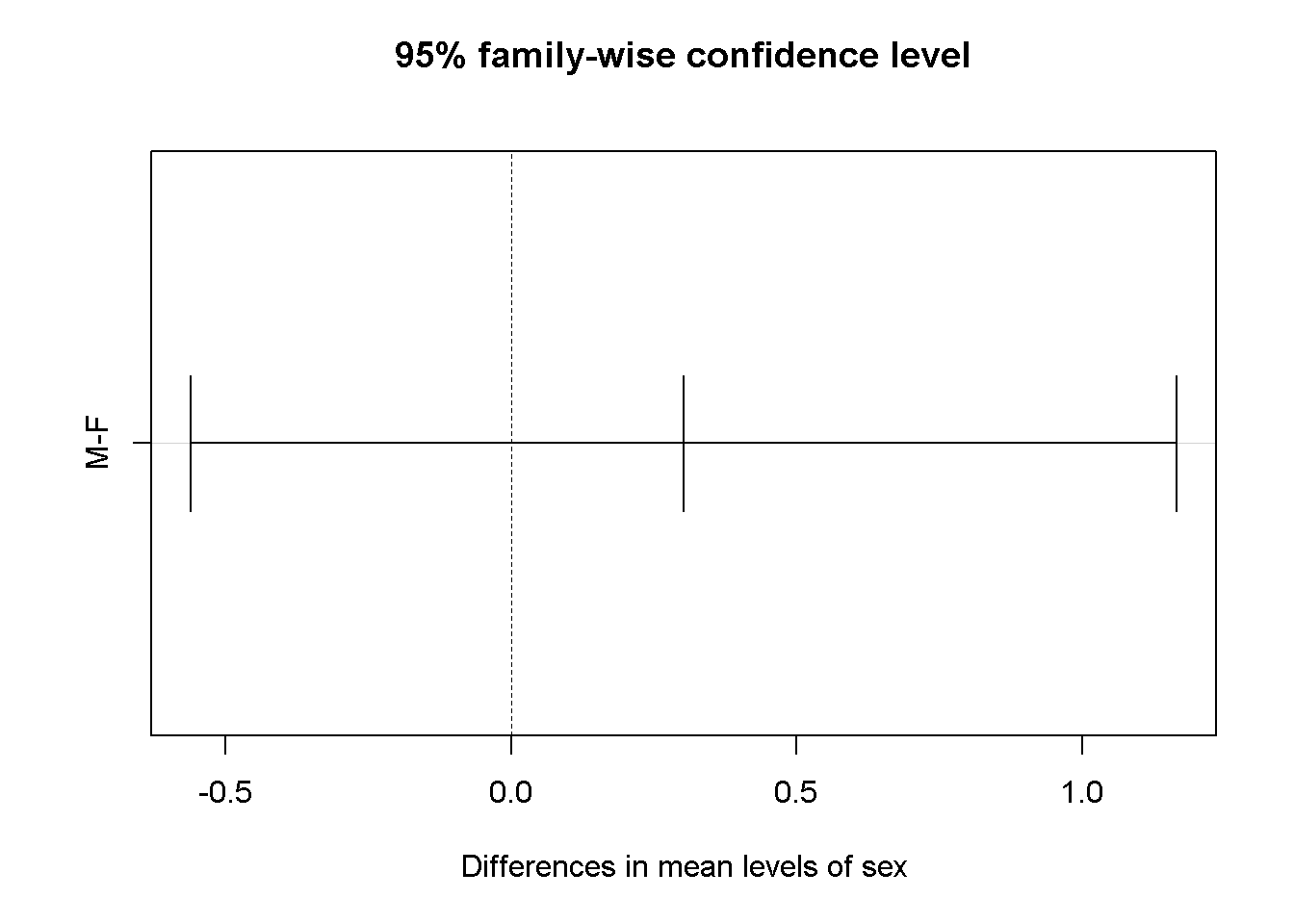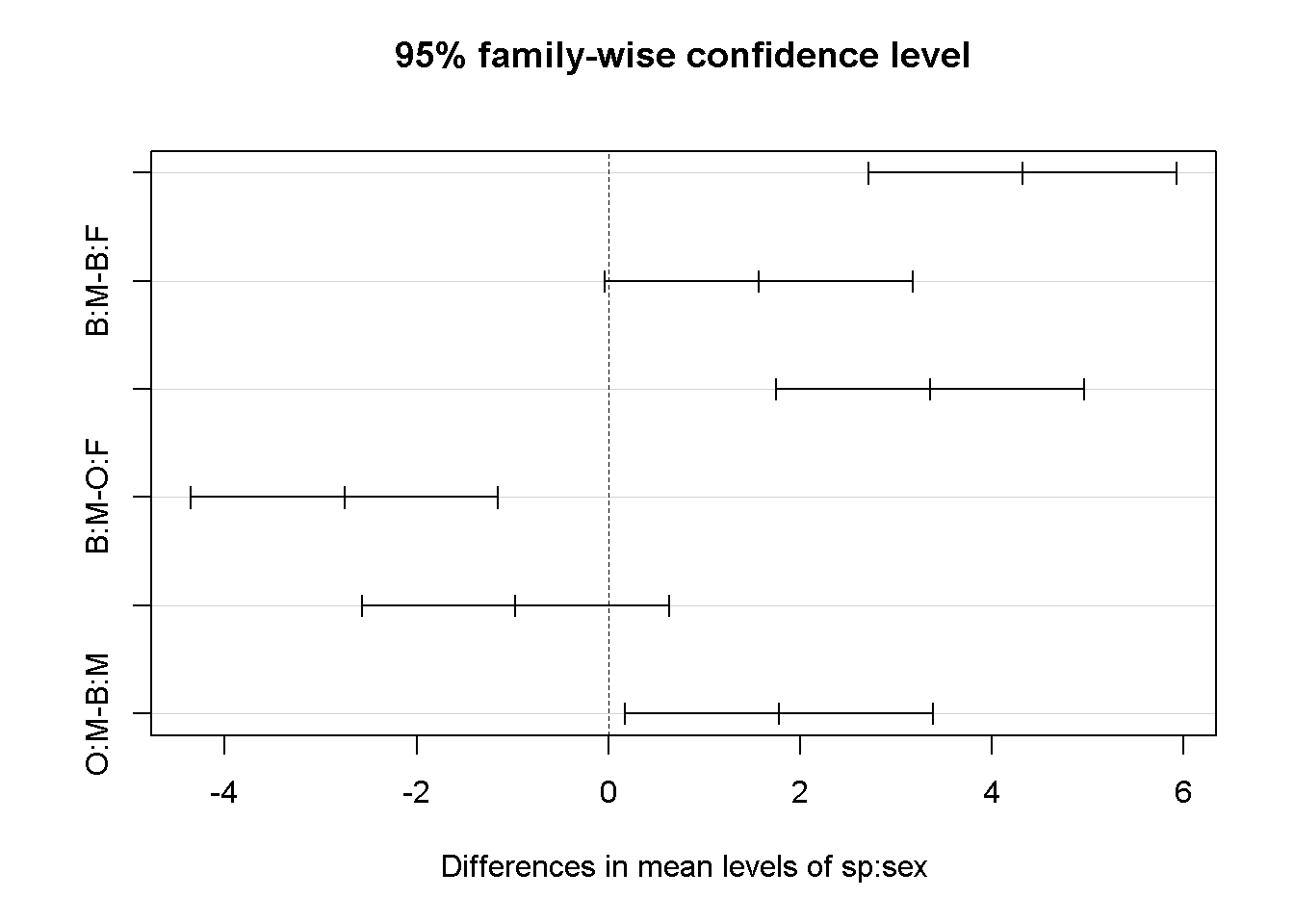plotTukeysHSD(crab.Tukey)